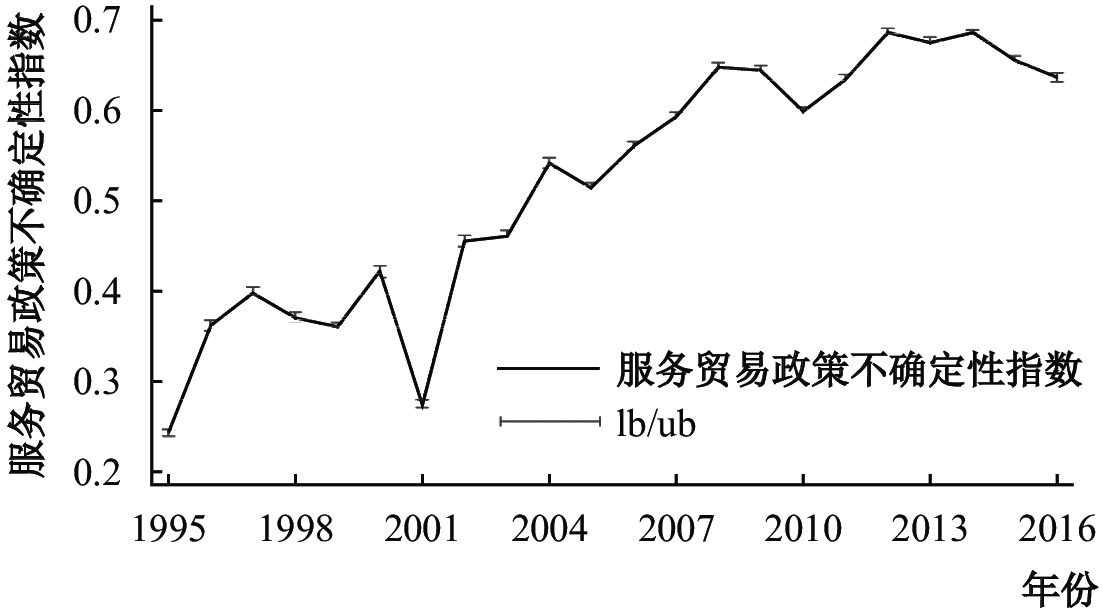﻿ 贸易政策不确定性冲击的贸易偏向效应研究《上海财经大学学报》
2022第24卷第4期

1.南京财经大学 国际经贸学院, 江苏 南京 210023;
2.南京邮电大学 经济学院, 江苏 南京 210023

2008年美国次债危机之后，全球经济发展模式亟待调整，贸易政策不确定性迅速上升。现有研究表明，贸易政策不确定性的上升会抑制货物贸易(毛其淋，2020；Handley, 2014; Handley和Limão,2017)，但是鲜有文献考察贸易政策不确定性与服务贸易的关系。事实上，在全球产业结构日趋“软化”的背景下，服务贸易已经成为各国经济景气和增长潜能的风向标。如果忽略贸易政策不确定性对服务业出口的影响，就无法为服务经济时代的全球经济增长和贸易发展提供有益的建议。贸易政策不确定性上升会导致货物贸易下降，为了应对冲击，各国必然会大力发展服务贸易，从而为服务业出口提供了窗口期。

(二) 贸易政策不确定性、生产率和服务业出口

(三) 贸易政策不确定性、创新和服务业出口

(四) 贸易政策不确定性、时间和服务业出口

 $sexpor{t_{ijt}} = \frac{{gd{p_{it}}\times gd{p_{jt}}}}{{dis{t_{ij}}}}$ (1)

 $sexpor{t_{ijt}} = \frac{{gd{p_{it}}\times gd{p_{jt}}}}{{{{(dis{t_{ij}})}^{1 + \varepsilon }}}}$ (2)

 $dis{t_{ijt}} = \sqrt {\mathop \prod \limits_k d_{ijt}^k} ,k = geo,eco,cul,pol,tec$ (3)

 $dist_{_{ijt}}^{tpu} = tp{u_{ijt}}^x$ (4)

 $sexpor{t_{ijt}} = \frac{{gd{p_{it}}\times gd{p_{jt}}}}{{{{(\sqrt {d_{ijt}^{geo}\times d_{ijt}^{tpu}} )}^{1 + \varepsilon }}}}$ (5)

 $sexpor{t_{ijt}} = \frac{{gd{p_{it}}\times gd{p_{jt}}}}{{{{(\sqrt {d_{ijt}^{geo}\times d_{ijt}^{tpu}\times t_{ij}^{^{\upsilon - \zeta }}} )}^{1 + \varepsilon }}}}$ (6)

 $\ln sexpor{t_{ijt}} = {\mathop {\theta } \limits^ \wedge}_0 + {\mathop \theta \limits^ \wedge }_1\ln gd{p_{it}} + {\mathop \theta \limits^ \wedge }_2 \ln gd{p_{jt}} - \frac{{1 + \varepsilon }}{2}\ln d_{ijt}^{geo} - \frac{{(1 + \varepsilon )x}}{2}\ln tp{u_{ijt}} - \frac{{(1 + \varepsilon )(\upsilon - \zeta )}}{2}\ln t_{ij}^{^{\upsilon - \zeta }}$ (7)

 $\ln sexpor{t_{ijt}} = {\mathop \theta \limits^ \wedge }_0 + {\mathop \theta \limits^ \wedge }_1\ln pgd{p_{it}} + {\mathop \theta \limits^ \wedge }_2\ln pgd{p_{jt}} - \frac{{1 + \varepsilon }}{2}\ln d_{ijt}^{geo} - \frac{{(1 + \varepsilon )x}}{2}\ln tp{u_{ijt}} - \frac{{(1 + \varepsilon )(\upsilon - \zeta )}}{2}\ln t_{ij}^{^{\upsilon - \zeta }} + {\rho _{ijt}}$ (8)

Cafiso(2011)进一步指出，服务品出口也需要考虑边界效应。因此，本文将地理边界和文化边界纳入服务贸易的计量方程：

 $\begin{split} \ln sexpor{t_{ijt}} = & {\mathop \theta \limits^ \wedge }_0 + {\mathop \theta \limits^ \wedge }_1\ln pgd{p_{it}} + {\mathop \theta \limits^ \wedge }_2\ln pgd{p_{jt}} - \frac{{1 + \varepsilon }}{2}\ln d_{ijt}^{geo} - \frac{{(1 + \varepsilon )x}}{2}\ln tp{u_{ijt}} \\ & - \frac{{(1 + \varepsilon )(\upsilon - \zeta )}}{2}\ln t_{ij}^{^{\upsilon - \zeta }} + {\mathop \theta \limits^ \wedge }_3languag{e_{ij}} + {\mathop \theta \limits^ \wedge }_4borde{r_{ij}} + {\rho _{ijt}} \end{split}$ (9)

 $\begin{split} \ln sexpor{t_{ijt}} = & {{\hat \theta }_0} + {{\hat \theta }_1}\ln pgd{p_{it}} + {{\hat \theta }_2}\ln pgd{p_{jt}} - \frac{{1 + \varepsilon }}{2}\ln d_{ijt}^{geo} - \frac{{(1 + \varepsilon )x}}{2}\ln tp{u_{ijt}} - \frac{{(1 + \varepsilon )(\upsilon - \zeta )}}{2}\ln t_{ij}^{\upsilon - \zeta } \\ & + {{\hat \theta }_3}languag{e_{ij}} + {{\hat \theta }_4}borde{r_{ij}} + {{\hat \theta }_5}NAFTA + {{\hat \theta }_6}OECD + {{\hat \theta }_7}AS EAN + {\rho _{ijt}} \\[-12pt] \end{split}$ (10)

(二) 变量定义

 $TPU=1-\left(\frac{{\tau }_{B}}{{\tau }_{MFN}}\right)^{-\sigma }$ (11)

 $TPU=1-\left(\frac{{\tau }_{2v}}{{\tau }_{mv}}\right)^{-\sigma }$ (12)

 $antidum{p_{ijt}} = antidum{p_{ijt}} + \sqrt {{{(antidum{p_{ijt}})}^2} + 1}$ (13)

 $TP{U}_{ijt}=\left[1-\left(\frac{{\tau }_{2v}}{{\tau }_{mv}}\right)^{-\sigma }\right]\left[antidum{p}_{ijt}+\sqrt{{(antidum{p}_{ijt})}^{2}+1}\right]$ (14)图 1 1995年至2016年全球贸易政策不确定性指数(TPU)

3. 空间效应。本文对空间效应的测度采用与Kimura和Lee(2006)相同的方法，即用两国首都之间的距离来表示

4. 时间效应。本文对时间的测度采用两国的时差来表示，考虑到地球的球形特征，时差采用相对概念(时差的绝对数值控制在12小时以内)。本文对于时间的选择采用格林威治平均时间(Greenwich Mean Time，GMT)。

 变量名称 观测值 均值 标准差 最小值 最大值 lnsexport 255 420 11.7187 7.1856 0 24.8594 lnpsexport 255 420 15.0645 3.1541 0 24.6047 lncsexport 255 420 13.5598 3.5485 0 24.0962 lngsexport 255 420 11.6691 2.9679 0 22.5733 lnTPU 255 420 0.5306 0.3983 0 31.9006 lnhgpd 255 420 22.0228 1.4009 18.6937 26.5259 lnfgpd 255 420 19.5713 2.2673 11.9429 28.997 lndis 255 420 8.7193 0.7875 0 9.8921 lnt 255 420 1.2485 0.8866 0 2.4849 border 255 420 0.0144 0.1191 0 1 language 255 420 0.0465 0.2106 0 1 NAFTA 255 420 0.0005 0.0227 0 1 Asean 255 420 0.0007 0.0262 0 1 OECD 255 420 0.0449 0.2072 0 1 注：由Stata15计算得出，保留4位有效数字。

 GLS PPML 方程（1） 方程（2） 方程（3） 方程（4） lnsexport lnsexport lnsexport lnsexport lnTPU 0.0175***(0.00447) 0.0473***(0.00641) lnTPU×lnt −0.0198***(0.00245) −0.0416***(0.00399) lnt 0.0200***(0.00276) lnhgpd 1.021***(0.0236) 0.0573***(0.000759) 0.0574***(0.000757) 0.0571***(0.000757) lnfgpd 1.024***(0.0161) 0.0795***(0.000501) 0.0797***(0.000504) 0.0797***(0.000504) lndis −1.055***(0.0526) −0.0679***(0.00153) −0.0604***(0.00180) −0.0660***(0.00200) border 0.249(0.331) −0.0258***(0.00662) −0.0201***(0.00670) −0.0227***(0.00672) language 1.178***(0.178) 0.0903***(0.00474) 0.0932***(0.00476) 0.0925***(0.00476) nafta −1.406(2.376) −0.0915*(0.0476) −0.0944**(0.0480) −0.0960**(0.0476) asean 0.0915(1.274) 0.0313(0.0395) 0.0338(0.0396) 0.0438(0.0398) OECD 1.842***(0.100) 0.149***(0.00315) 0.147***(0.00323) 0.142***(0.00336) Constant −20.76***(0.723) 0.286***(0.0231) 0.218***(0.0253) 0.246***(0.0257) Wald chi2 9509 Pseudo log-likelihood −697607 −697498 −697430 Observations 255 420 255 420 255 420 255 420 R-squared 0.4432 0.2197 0.2199 0.220 注：(1)实证结果均由Stata15计算并整理得出，***、** 、* 分别表示1%、5%、10%的显著性水平，下同。GLS聚类到国家层面，PPML无法进行聚类，圆括号中的数字为双尾检验的t值。(2)hgdp表示母国人均GDP，pgdp表示伙伴国人均GDP。(3)方程（1）是采用随机效应GLS的回归结果，方程（2）−（4）是采用泊松伪极大似然估计的回归结果。

(二) 贸易政策不确定性对服务业出口的影响机制

 方程（1） 方程（2） 方程（3） 生产率 制度 创新 lnsexport lnsexport lnsexport lnTPU 0.0257***(0.00683) 0.560***(0.0378) 0.103***(0.0129) lnt 0.0212***(0.00284) 0.0149***(0.00281) 0.0209***(0.00275) lnTPU×lnt −0.0437***(0.00414) −0.0355***(0.00409) −0.0317***(0.00396) tfp 0.0752***(0.0156) lnTPU×tfp 0.0456***(0.00195) lnregulation 0.215***(0.0110) lnTPU×lnregulation −0.275***(0.0192) lnpatent −0.0105***(0.00139) lnTPU×lnpatent −0.0117***(0.00172) 控制变量 Y Y Y Pseudo log-likelihood −696828.88 −697019.4 −696472.06 Observations 165 666 165 666 165 666 R-squared 0.222 0.221 0.223 注：方程（1）中tfp表示全要素生产率，我们采用世界银行提供的跨国GDP(2010年不变价美元)、固定资本形成总额(2010年不变价美元)和劳动力总数，采用DEA进行计算。方程（2）中regulation表示制度，该数据来源于加拿大佛雷泽研究院每年公布的贸易自由化指数。Kimura和Lee(2006)、毛艳华和李敬子(2015)对服务业出口的分析都采用了该数据库的指标。①方程（3）中patent表示创新能力，利用世界银行提供的非居民专利数表示。
(三) 细分贸易种类

(四) 稳健性检验

1、稳健性检验Ⅰ：更换弹性系数。考虑到不同的替代弹性会影响TPU的数值，Handley(2014)将 $\sigma$ 设定为4。本文借鉴其做法，分别将替代弹性设置为4、5、8和10，考察不同的替代弹性下TPU对服务业出口的影响。实证结果显示：随着服务品替代弹性的增加，贸易政策不确定性对服务业出口额的偏回归系数逐步下降；但是在不同替代弹性的影响下，TPU和服务业出口都表现为显著的正向关系。这意味着TPU对服务业出口的正向关系不会受到不同替代弹性的影响。此外，时差对服务业出口的正向关系依旧显著，并且TPU和时差交互项的系数显著为负。这表明，改变服务品的替代弹性之后，贸易政策不确定性与服务业出口之间的正向关系依旧显著成立；并且TPU和时差的交互项系数依旧为负。因此本文的回归结果不会受到测度方法的影响，结果稳健。

 GLS PPML 生产性服务业 出口 消费性服务业 出口 公共服务业 出口 生产性服务业 出口 消费性服务业 出口 公共服务业 出口 方程(1) 方程(2) 方程(3) 方程(4) 方程(5) 方程(6) lnpsexport lncsexport lngsexport lnpsexport lncsexport lngsexport lnTPU 1.297***(0.0173) 1.225***(0.0209) 0.881***(0.0224) 0.0970***(0.00287) 0.0958***(0.00305) 0.103***(0.00348) lnt 0.161***(0.0288) 0.261***(0.0331) 0.0149 (0.0329) 0.00162*(0.00122) 0.00881***(0.00137) 0.0106***(0.00157) lnTPU×lnt −0.164***(0.0105) −0.148***(0.0126) −0.0885***(0.0135) −0.0131***(0.00195) −0.0121***(0.00211) −0.00 (0.00241)812*** 控制变量 Y Y Y Y Y Y Wald chi2 32213 25462 9067 Pseudo log-likelihood −311149 −308634 −293042 Observations 255 420 255 420 255 420 255 420 255 420 255 420 R-squared 0.3505 0.3787 0.2288 0.648 0.636 0.478

2、稳健性检验Ⅱ：更换被解释变量。为了解决可能的内生性问题，本文分析了服务业出口内生的需求冲击。需求冲击是指由于不可观测的需求因素，导致对消费性服务品的进口需求增加。为了解决内生性问题，本文参考Hummels等(2014)进行工具变量检验。Hummels 等(2014)在研究外包对丹麦工人收入的影响中，将全球服务业出口总额与本国服务业出口额的差值作为本国服务业出口的工具变量。其原理为：世界总出口(剔除本国)的增长可以反映出同期需求的变化，并且该变量不会受到国内因素的影响。因此，本文第二个稳健性检验是更换被解释变量，利用伙伴国同期总的服务进口额减去母国服务出口额。为了进一步避免解释变量和被解释变量之间可能存在的内生性问题，本文借鉴Behrens等(2011)、Guillin(2013)以及Ariu(2016)解决内生性问题的处理方法，对被解释变量进行差分处理： $d\ln sexpor{t_{ijt}} = \ln sexpor{t_{ijt}} - \ln sexpor{t_{ijt - 1}}$ ，经过差分之后的被解释变量消除了时间趋势，较好地克服了内生性问题。此外，本文也将双边国家的GDP取差分。

① 本文将服务贸易占总贸易比重上升的现象称之为贸易的结构性偏向。

② Francois等(2015)发现全球化和区域化改变了中间品贸易的复杂性，使服务环节内嵌于生产活动中。在总量贸易中，服务贸易的占比为18.6%，但在附加值贸易中，这一比例上升至50%。

③ 如企业可以利用时差将部分活动外包到其他国家，以降低成本。

④ 智利在2009年加入OECD，在2009年之间该国的“OECD”设为0，之后设为1。

⑤ 数据来源：http://tariffdata.wto.org/ReportersAndProducts.aspx。

⑥ 我们将设定不同的σ，进行稳健性检验。

⑦ Crowley等(2018)认为关税只能作为考察TPU的一个维度，测度TPU还需要考虑非关税因素，他们分析了反倾销调查对出口的影响。

⑧ 该数据来源于世界银行临时贸易壁垒数据库（Temporary Trade Barriers Database ,TTBD）, http://econ.worldbank.org/ttbd/。

⑨ 距离的计算由https://www.timeanddate.com/worldclock/distance.html获取。

⑩ 考虑到存在不随时间变化的时差变量和地理变量，无法采用固定效应。

⑪ 类似Melitz（2003）阐述的自选择机制。

⑫ 版面所限，实证结果未列示，有需要可联系作者。

⑬ 数据来源于：https://www.fraserinstitute.org/studies/economic-freedom。

⑭ 生产性服务贸易包括保险服务、计算机和信息服务、建筑业、运输服务、金融服务、其他商业服务、特许使用和许可费；消费性服务贸易包括旅游服务、个人文化娱乐服务；公共服务贸易为政府服务。

⑮ 此外，Chen 等(2017)在分析中间品贸易自由化对中国工人技能升水的影响时，也采用一阶差分解决内生性问题。Fiorini等(2018)在分析服务业改革对制造业就业的影响时，也将被解释变量取差分进行回归。